### The Agni Purana

by N. Gangadharan | 1954 | 360,691 words | ISBN-10: 8120803590 | ISBN-13: 9788120803596

This page describes Pancanga: The Almanac which is chapter 122 of the English translation of the Agni Purana, one of the eighteen major puranas dealing with all topics concerning ancient Indian culture, tradition and sciences. Containing roughly 15,000 Sanskrit metrical verses, subjects contained in the Agni-Purana include cosmology, philosophy, architecture, iconography, economics, diplomacy, pilgrimage guides, ancient geography, gemology, ayurveda, etc.

# Chapter 122 - Pañcāṅga: The Almanac

[Note: Pañcāṅga or the five aspects of reckoning time for each day, computed and recorded in Hindu almanacs are:

1. Vāra (day of the week),
2. Tithi (lunar day),
3. Nakṣatra (the Constellation through which the moon moves),
4. Yoga (Sum of the longitudes of the Sun and the Moon), and
5. Karaṇa (half-tithi).

All these reckonings have religious and ritualistic significances.

The verses in this chapter are all of a highly cryptic nature and, like philosophical aphorisms, imply much more than what is actually expressed, and can be correctly understood only with suitable explanations. While the implied information is given brackets in the translation, an example is worked out in the Notes illustrating the working, step by step.]

## God Agni said:

1a. Time is (reckoned by) the accumulated number of years, (months etc., from the epoch up to the point of time under consideration). I shall set forth the calculation involved in reckoning time.

1b. The accumulated (i.e. elapsed) number of years (up to the required point of time) is to be multiplied by 12 (arka) and the (number of months elapsed in the current year from) Citrā is to be added to the product)

2a. The sum obtained is doubled and placed at two places. To one is added 4 (veda) and to the other 865 (pañcāṅgāṣṭa). (The latter figure is to be divided by 60 and the quotient added to the first, while the remainder is kept as the second figure). The resultant is to be called ‘guṇa’.

2b. (The guṇa) is placed at three places (one below the other, in the serpentine fashion, each being written one step to the right of the preceding one). The ‘middle’ is multiplied by 8 (vasu) and the product again multiplied by 4 (veda). (The columns are to be added up.) and again written as ‘upper’, ‘middle’ and ‘lower’.

3. Subtract 398 (aṣṭa-randhra-agni) from the lower and 87 (saika-rasāṣṭaka) from the ‘middle’. Divide (the ‘middle’ and the ‘lower’) by 60 and add the quotients to the preceding, (keeping the remainders in their places).

4a. Vāra-tithi correction. The first (i.e. ‘upper’) when divided by 7 will give the week-day constant for tithi. (The quotient is to be rejected as of no more use.) The resultant is to be used as the Vāra-correction for tithi-nāḍikās.

4b-5. Nakṣatra-Yoga constants. The ‘guṇa’ derived above is to be multiplied by 2, and 3 subtracted from the second figure. Guṇa is set down before the result (in the serpentine fashion). 30 (kha-rāma) is set down below the last figure, and 6, 12 and 8 (rasa-arka-aṣṭa), respectively, below the three figures, (and the columns added up and elevated by dividing by 60). Divide the first figure by 28 and place it below the ‘correction for tithi’ (obtained in verse 4a). (add and take the result as a second ‘upper’).

6-8a. The ‘guṇa’ is halved, 3 subtracted (from its second figure) and the whole expression multiplied by 2. The first figure here is multiplied by 11 (rudra); the second figure is increased by 1 and divided by 39, the quotient being subtracted from the first figure and the remainder kept in its place. The resultant is termed madhya. Subtract 22 from the first figure and divide it by 60; the remainder is deductive; the quotient is added to the (second) ‘upper’ (of verses 4b-5). The first figure is divided by 27 and the remainder set down in its place. The resultant expression is the constant for the correction of nakṣatra and yoga.

8b-9a. Nakṣatra. For the calculation of tithi, there is a monthly constant, being 2 piṇḍas (i.e. whole units) and 32 nāḍikās (which has to be added to the tithi correction contained in verses 4a). Similarly, for the nakṣatra, there is a monthly constant, being 2 nakṣatras and 11 nāḍikās, (which, when added to the nakṣatra correction of verse 8a, will give the elapsed nakṣatra and the nāḍikās gone in the current nakṣatra.)

9b-10. Weekday and commencing point of Tithi. Add the tithi-correction above (to the vārādi-tithi correction got in verse 4a), placing the tithi number below the vāra number. Divide by 7 (if vāra plus tithi exceeds 7); the remainder will give the elapsed week-day counted from Sunday, and the nāḍikās gone in the next day at the point of the commencement of the relevant tithi) In the case of tithis after adding the complete units (Piṇḍakas), the sum should be divided by 14 (if the sum exceeds 14, and the remainder taken as the tithi.)

11-14a. True-Tithi correction. The correction, in nāḍikās, for the fourteen tithis would be, in order, minus, plus, plus, minus, (minus and so on). While the correction for the 14th tithi is zero), the correction for the thirteenth and the first is 5 (vināḍikās) (each, minus and plus, respectively), that for the 12th and the 2nd, 10 (vināḍikās), that for the 11th and 3rd, 15 (vināḍikās), that for the 10th and 4th, 19 (vināḍikās), that for the 9th and 5th, 22 (vināḍikās), that for the 8th and the 6th, 24 (vināḍikās), and that for the 7th, 25 (vināḍikās). These khaṇḍakas (correction-bits) are to be applied appropriately to the piṇḍakas (full units).

14b-17a. Vikālā correction. In the case of (the three), Karkaṭaka, (Siṃha and Kanyā), divide the rāśis, respectively, by 6 (ṛtu), 4 (veda) and 3 (traya); in the case of Tulā, (Vṛścika and Dhanus), divide, respectively, in the reverse, i.e. by 3, 4 and 6; in the case of Makara, (Kumbha and Mīna), respectively, by 3, 4 and 6; and in the case of Meṣa, (Ṛṣabha and Mithuna), divide, respectively, in the reverse, i.e. by 6, 4 and 3. The correction, in vikalās, which is positive in the case of the three, Meṣa etc., are 50 (kha-iṣu), 40 (kha-yuga) and 12 (mitra); in the case of the three, Karkaṭaka etc., it is in the reverse order, (i.e. 12, 40 and 50, but positive); in the case of the three, Tula etc., (it is 50, 40 and 12), negative; (and, in the case of the three, Makara etc., it is 12, 40 and 50, negative).

17b-19a. Application of the vikalā correction. The vikalā correction is to be applied to the tithi multiplied by 4. Multiply their eleven vikalās by the difference in liptās (i.e. kalās) between the elapsed and to-elapse portions of the tithi and divide by 60. If the elapsed portion is less than/the portion to-elapse, treat the correction as positive even if it be negative and or positive, retain it as positive; while, in the case of the portion toelapse being greater, the reverse is the case (i.e. the correction is to be taken as negative both if it is negative or positive).

19b-21a. Further correction to the Tithi. Double (?Treble) (the nāḍikās of) the tithi and subtract from it one-sixth of (the product). Apply to it the tithi-nāḍikās obtained for the sun in the reverse order, and subtract the result from 60; the true nāḍis of the tithi would be obtained. If not subtractible, add 60 and subtract; if more than 60, reduce it by 60 and subtract.

21b-22. Toga. The tithi is associated with the constellation The tithi multiplied by 4 and a third (of the tithi) added to it. Apply to it the negative correction. (By dividing it by 27, the yoga elapsed and the nāḍikās in the current yoga are obtained.) True tithi has be to be used as the means for calculating the yoga.

23a. The yoga is, indeed, got also by adding (the longitudes of) the sun and the moon (in kalās and dividing by 800).

23b-24a. Karaṇa. (The number of) the tithi reduced by 1 and multiplied by 2, and the product divided by 7, gives the karaṇa of day-time. The tithi-number multiplied by 2 and the product reduced by 1 and divided by 6 (kṛta) gives the karaṇa of the night.

24b-c. The karaṇa of the end (i.e. second half) of the 14th tithi of the dark fortnight is called Śakuni; (that of the first half of the full moon day is called Catuṣpada; that of the end (i.e. second half of the full moon day) is called Ahi (Nāga); and that of the beginning (i.e. first half) of the prathamā (of the bright fortnight) is called Kiṃstughna.

## Footnotes and references:

:

Any epoch or starting point in time might be adopted, like, for instance the Kalpa or the beginning of creation, the Caturyuga, Kaliyuga etc. or any of the eras like the Śaka.

Let the point of time taken for consideration, for which the Pañcāṅga is required be Śaka 10 (elapsed), in the month of Vaiśākha, 1st tithi of the bright fortnight.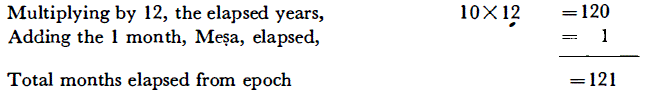:

Multiplying the months from epoch by 2 (i.e. 121 X 2 = 242) and placing them in two places: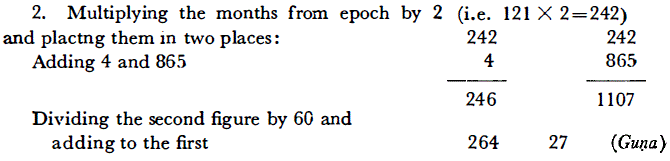: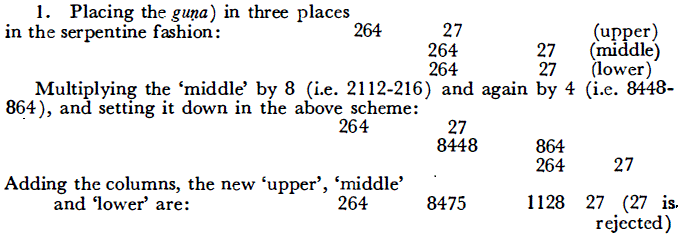: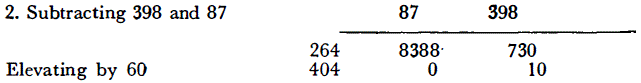:

Dividing the ‘upper’ by 7 for week-days and rejecting the quotient, we get the vāra-correction for tithi as 5-0-10 .

: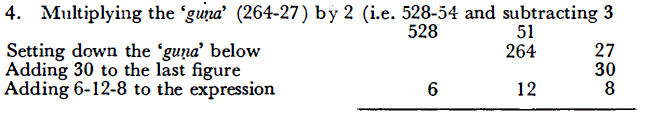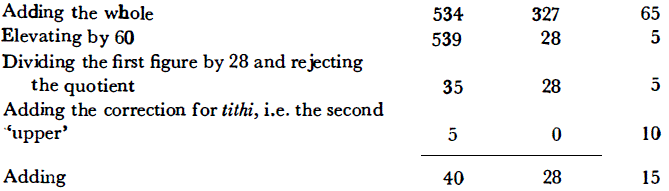:

The guṇa (264-27) is halved (132-14), 3 subtracted from the second figure (132-11) and multiplied by 2 (i.e. 264-22). The first figure is multiplied by 11 (i.e. 2904); to the second figure 1 is added (i.e. 23) and divided by 39 and the remainder (i.e. 23) is retained. This is termed the new 'middle'. Subtracting 22 from the first figure (i.e. 2882-23) and elevating by 60, we get 48-2-23.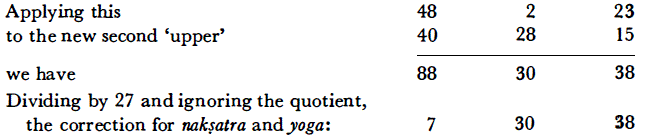:

Adding the tithi-constant (2-32-00) to the vāra-tithi correction (5-0-10), we have 7-32-10, being the vārādi (week, day etc.) correction for the point of time taken for consideration. For the nakṣatra of that point of time, add the nakṣatra constant (2-11-00) to the nakṣatra correction (got in verses 6-8a), i.e. 7-30-38, and we have the nakṣatra as 9-41-38. In other words, at the point of time taken, nine nakṣatras have passed by, and in the current 10th nakṣatra, maghā, it is 41 nāḍikās and 38 vināḍikās.

:

Adding the tithi constant (2-32-00) to the vāra-tithi correction (5-0-10). we get 7-32-10 for the week, day, which means that 7 days have elapsed as / counted from Sunday, and at the point of time in consideration, i.e. Śuklapratipad in Vaiśākha, it is Sunday at 32 nāḍikās and 10 vināḍikās (Mean).

:

The nāḍikā corrections enunciated are:

 Tithi Nāḍikā corr. Tithi Nāḍikā corr. 14 0 1 +5 13 -5 2 +10 12 -10 3 +15 11 -15 4 +19 10 -19 5 +22 9 -22 6 +24 8 -24 7 +25

:

The vikālā corrections enunciated are:

 Month Divisor Corr. Month Divisor Corr. Karkaṭaka 6 +12" Makara 6 -12" Siṃha 4 +40" Kumbha 4 -40" Kanyā 3 +50" Mīna 3 -50" Tulā 3 +50" Meṣa 3 -50" Vṛścika 4 +40" Ṛṣabha 4 -40" Dhanus 6 +12" Mithuna 6 -12"

:

For the third tithi correction, multiply the tithi-nāḍikās, 32-10 (of verses 9b-10) by 3 (i.e. 96-30), and deduct a sixth thereof (i.e. 16-5), resulting in 80-25. Adding to this the tithi-nāḍikās (32-10), we get 112-35. The correction for one tithi (viz. 5 nāḍikās, of verse 11-14a) being applied negatively, we get 107-35. Dividing it by 60, the true nāḍikās in the current tithi is got at 47-35.

:

Yogas form variable divisions of time during which the joint motion of the Sun and the Moon in longitude is 13° 20' or 800 kalās. In a cycle there are 27 yogas, some of them being considered as auspicious and others inauspicious. Their significances are taken due note of in deciding upon the times for rituals and domestic ceremonies. The 27 yogas are:

1. Viṣkambha,
2. Prīti,
3. Āyuṣmān,
4. Saubhāgya,
5. Śobhana,
6. Atigaṇḍa,
7. Sukarmā,
8. Dhṛti,
9. Śūla,
10. Gaṇḍa,
11. Vṛddhi,
12. Dhruva,
13. Vyāghāta,
14. Harṣaṇa,
15. Vajra,
16. Siddhi,
17. Vyatīpāta,
18. Varīyān,
19. Parigh,
20. Śiv,
21. Siddh,
22. Sādhy,
23. Śubh,
24. Śukr,
25. Brahm,
26. Indr,
27. Vaidhṛt.

Multiplying the true tithi (viz. 47-35 of verses 19-20) by 4 (i.e. 190-20) and adding a third of the tithi (viz. 15-52), we get 206-12. Dividing by 27 (which is the total number of yogas in a cycle) and rejecting the quotient, we have 7-12. i.e. 7 yogas up to Sukarmā have gone by and, in the current 8thyoga, Dhṛti, 12 nāḍikās too are over.

:

In the alternative method of calculating the yoga, the longitudes of the Sun and the Moon are added and the sum divided by 800 (which is the said sum in terms of six kalās). The quotient will give the number of the yogas elapsed and the remainder the nāḍikās etc. elapsed in the current yoga.

:

The Karaṇa is a measure of time extending over half a tithi, having astrological significance. They carry eleven names, being: (1) Bava, (2) Bālava, (3) Kaulava, (4) Taitila, (5) Gara., (6) Vaṇija, (7) Viṣṭi, (8) Śakuni, (9) Catuṣpada, (10) Nāga and (11) Kiṃstughna. A cycle consists of 60 karaṇas (during the 30 tithis or 60 half-tithis). The last four karaṇas occur only once and occupy the four half-tithis from the second half of the 14th of the dark fortnight to the first half of the/1st tithi of the bright fortnight, and, so, are called sthira or ‘fixed’ karaṇas, while the other seven karaṇas occupy the remaining 56 half-tithis, by repeating themselves eight times, and, so, are called cala or ‘moving’ karaṇas.

Like what you read? Consider supporting this website: